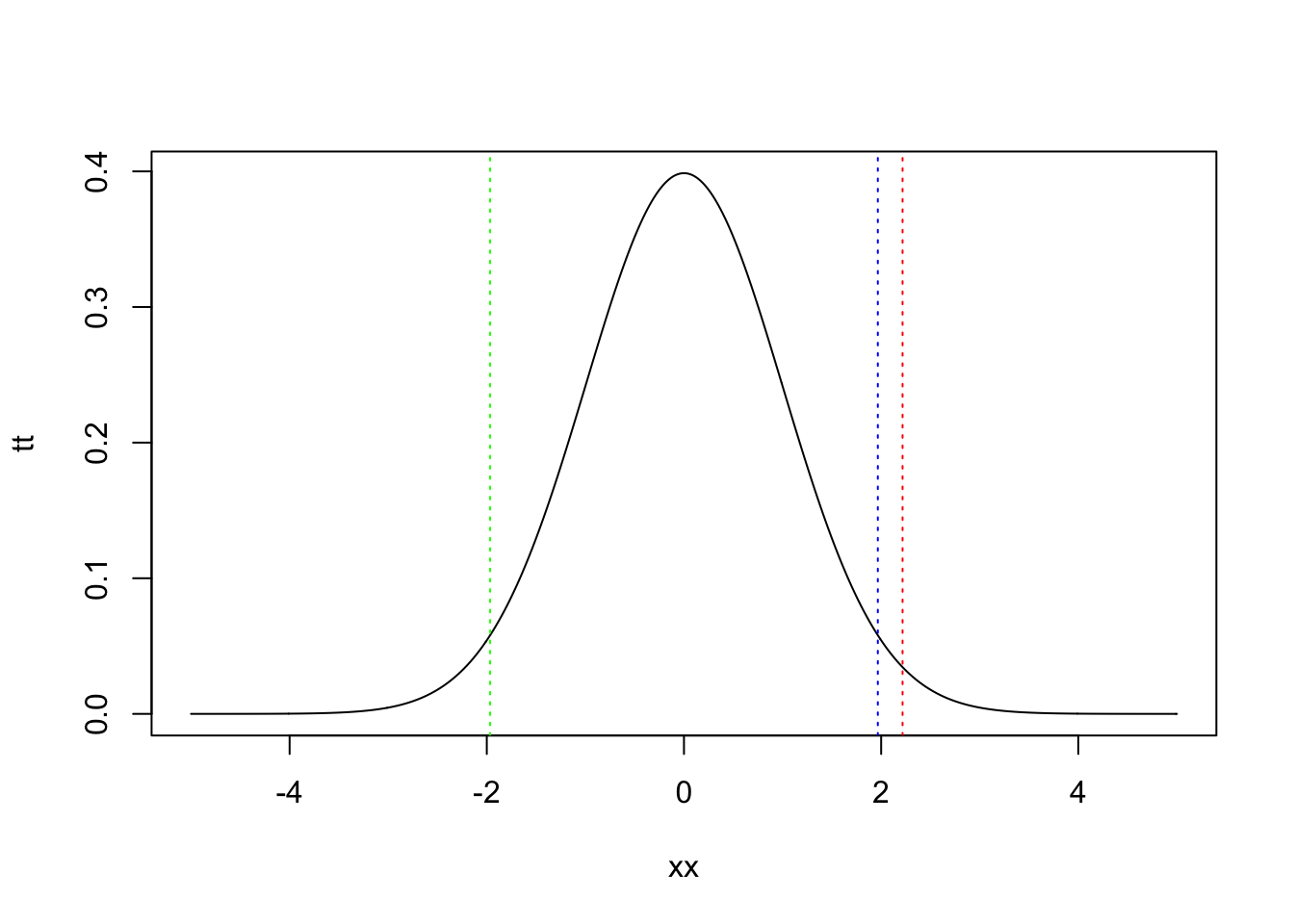## R Markdown

Prepare data.

pheno <- read.csv("RiceDiversityPheno.csv")
line.pheno <- merge(line, pheno, by.x = "NSFTV.ID", by.y = "NSFTVID")
data <- data.frame(
height = line.pheno$Plant.height, flower = line.pheno$Flowering.time.at.Arkansas)
data <- na.omit(data)
x <- data$flower y <- data$height

Calculate regression coefficient.

n <- length(x)
ssx <- sum(x^2) - n * mean(x)^2
ssxy <- sum(x * y) - n * mean(x) * mean(y)
b <- ssxy / ssx
b
##  0.6728746
m <- mean(y) - b * mean(x)
m
##  58.05464

Calculate MSE and SSX.

ssr <- b * ssxy
ssr
##  26881.49
ssy <- sum(y^2) - n * mean(y)^2
sse <- ssy - ssr
sse
##  133903.2
mse <- sse / (n - 2)
mse
##  360.9251

test $$H_0: \beta_0 = 0.5$$.

t.value <- (b - 0.5) / sqrt(mse/ssx)
t.value
##  2.217253
2 * (1 - pt(t.value, n - 2))
##  0.02721132

Draw a graph of $$t$$-distribution to which $$\frac {b - 0.5} {\sqrt{MSE/SSX}}$$ with $$n - 2$$ degree of freedom follows.

xx <- seq(-5, 5, 0.01)
tt <- dt(xx, n - 2)
plot(xx, tt, type = "l")
# calculate the 100 * (1 - alpha/2) percentile
t.975 <- qt(1 - 0.025, n - 2)
t.975
##  1.966379
# calculate the 100 * alpha / 2 percentile
t.025 <- qt(0.025, n - 2)
t.025
##  -1.966379
# the above value can be calculated also as
- qt(1 - 0.025, n - 2)
##  -1.966379
# this is because the shape t-distribution is symmetric
# draw lines of the bounds
abline(v = t.025, col = "green", lty = "dotted")
abline(v = t.975, col = "blue", lty = "dotted")
# draw the line of t.value
abline(v = t.value, col = "red", lty = "dotted")The range from the green line to the blue line is the range in which the t-distribution has a value at the 95% probability. The red line is out of the range.

Next, calculate the range in which $$beta_0$$ is inclouded at the 95% probability, given the 2.5 percentile and 97.5 percentile of the $$t$$ distribution. When the $$beta_0$$ is included in the range, the following equation holds. $t_{n-2, 0.025} \le \frac {b - \beta_0} {\sqrt{MSE/SSX}} \le t_{n-2, 0.975}$ Here, the 2.5 percentile equal to the 97.5 percentile multiplied by $$-1$$, then $- t_{n-2, 0.975} \le \frac {b - \beta_0} {\sqrt{MSE/SSX}} \le t_{n-2, 0.975}$ $- t_{n-2, 0.975} {\sqrt{MSE/SSX}} \le b - \beta_0 \le t_{n-2, 0.975} {\sqrt{MSE/SSX}}$ $-b - t_{n-2, 0.975} {\sqrt{MSE/SSX}} \le - \beta_0 \le -b + t_{n-2, 0.975} {\sqrt{MSE/SSX}}$ $b - t_{n-2, 0.975} {\sqrt{MSE/SSX}} \le \beta_0 \le b + t_{n-2, 0.975} {\sqrt{MSE/SSX}}$ From the above equation, the confidence interval of $$\beta_0$$ is obtained.